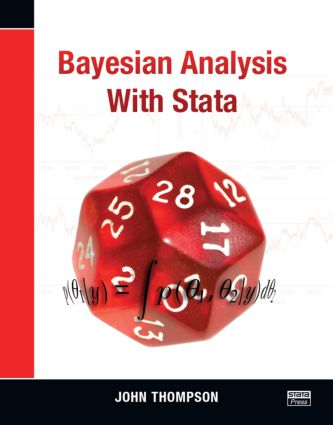# Bayesian Analysis with Stata

## 1st Edition

Stata Press

302 pages

##### Purchasing Options:\$ = USD
Paperback: 9781597181419
pub: 2014-05-06
SAVE ~\$15.19
\$75.95
\$60.76
x

FREE Standard Shipping!

### Description

Bayesian Analysis with Stata is written for anyone interested in applying Bayesian methods to real data easily. The book shows how modern analyses based on Markov chain Monte Carlo (MCMC) methods are implemented in Stata both directly and by passing Stata datasets to OpenBUGS or WinBUGS for computation, allowing Stata’s data management and graphing capability to be used with OpenBUGS/WinBUGS speed and reliability.

The book emphasizes practical data analysis from the Bayesian perspective, and hence covers the selection of realistic priors, computational efficiency and speed, the assessment of convergence, the evaluation of models, and the presentation of the results. Every topic is illustrated in detail using real-life examples, mostly drawn from medical research.

The book takes great care in introducing concepts and coding tools incrementally so that there are no steep patches or discontinuities in the learning curve. The book's content helps the user see exactly what computations are done for simple standard models and shows the user how those computations are implemented. Understanding these concepts is important for users because Bayesian analysis lends itself to custom or very complex models, and users must be able to code these themselves.

### Reviews

"… the first comprehensive guide to employing Bayesian methods using Stata statistical software. … until this book, there has been no unified presentation of how to implement Bayesian methods using Stata. … A nice feature of the book is the use of real data … I recommend it for Stata users who wish to employ Bayesian modeling within the Stata environment."

International Statistical Review, 2015

List of figures

List of tables

Preface

Acknowledgments

The problem of priors

Case study 1: An early phase vaccine trial

Bayesian calculations

Benefits of a Bayesian analysis

Selecting a good prior

Starting points

Exercises

Evaluating the posterior

Introduction

Case study 1: The vaccine trial revisited

Marginal and conditional distributions

Case study 2: Blood pressure and age

Case study 2: BP and age continued

General log posteriors

Adding distributions to logdensity

Changing parameterization

Starting points

Exercises

Metropolis–Hastings

Introduction

The MH algorithm in Stata

The mhs commands

Case study 3: Polyp counts

Scaling the proposal distribution

The mcmcrun command

Multiparameter models

Case study 3: Polyp counts continued

Highly correlated parameters

Case study 3: Polyp counts yet again

Starting points

Exercises

Gibbs sampling

Introduction

Case study 4: A regression model for pain scores

Conjugate priors

Gibbs sampling with nonstandard distributions

The gbs commands

Case study 4 continued: Laplace regression

Starting points

Exercises

Assessing convergence

Introduction

Detecting early drift

Detecting too short a run

Running multiple chains

Convergence of functions of the parameters

Case study 5: Beta-blocker trials

Exercises

Validating the Stata code and summarizing the results

Introduction

Case study 6: Ordinal regression

Validating the software

Numerical summaries

Graphical summaries

Exercises

Bayesian analysis with Mata

Introduction

The basics of Mata

Case study 6: Revisited

Case study 7: Germination of broomrape

Exercises

Using WinBUGS for model fitting

Introduction

Installing the software

Preparing a WinBUGS analysis

Case study 8: Growth of sea cows

Case study 9: Jawbone size

Advanced features of WinBUGS

GeoBUGS

Programming a series of Bayesian analyses

OpenBUGS under Linux

Debugging WinBUGS

Starting points

Exercises

Model checking

Introduction

Bayesian residual analysis

The mcmccheck command

Case study 10: Models for Salmonella assays

Residual checking with Stata

Residual checking with Mata

Exercises

Model selection

Introduction

Case study 11: Choosing a genetic model

Calculating a BF

Calculating the BFs for the NTD case study

Robustness of the BF

Model averaging

Information criteria

DIC for the genetic models

Starting points

Exercises

Further case studies

Introduction

Case study 12: Modeling cancer incidence

Case study 13: Creatinine clearance

Case study 14: Microarray experiment

Case study 15: Recurrent asthma attacks

Exercises

Writing Stata programs for specific Bayesian analysis

Introduction

The Bayesian lasso

The Gibbs sampler

The Mata code

Testing the code

Case study 16: Diabetes data

Extensions to the Bayesian lasso program

Exercises

A Standard distributions

References

Author index

Subject index

### About the Author

John Thompson is professor of genetic epidemiology at the University of Leicester and has many years experience working as a biostatistician on epidemiological projects.

### Subject Categories

##### BISAC Subject Codes/Headings:
MAT029000
MATHEMATICS / Probability & Statistics / General
MAT029010
MATHEMATICS / Probability & Statistics / Bayesian Analysis